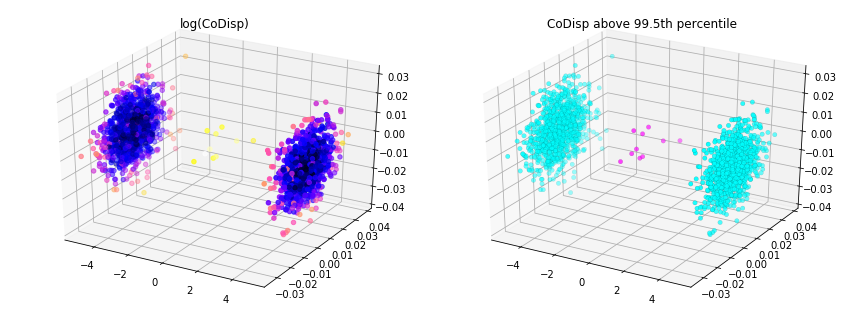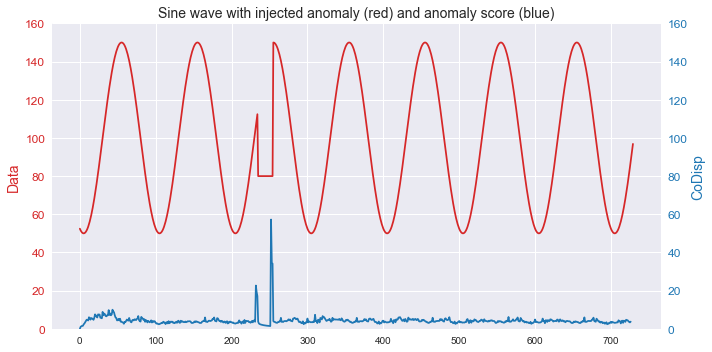# rrcf

🌲 Implementation of the Robust Random Cut Forest Algorithm for anomaly detection on streams

View the Project on GitHub kLabUM/rrcf

### Theory

• Related work and motivation
• Tree construction
• Insertion and deletion of points
• Anomaly scoring

### Basics

• RCTree data structure
• Modifying the RCTree
• Measuring anomalies
• API documentation
• Caveats and gotchas

### Examples

• Batch detection
• Streaming detection
• Analyzing taxi data
• Classification
• Comparison of methods

# rrcf 🌲🌲🌲

Implementation of the Robust Random Cut Forest Algorithm for anomaly detection by Guha et al. (2016).

S. Guha, N. Mishra, G. Roy, & O. Schrijvers, Robust random cut forest based anomaly detection on streams, in Proceedings of the 33rd International conference on machine learning, New York, NY, 2016 (pp. 2712-2721).

The Robust Random Cut Forest (RRCF) algorithm is an ensemble method for detecting outliers in streaming data. RRCF offers a number of features that many competing anomaly detection algorithms lack. Specifically, RRCF:

• Is designed to handle streaming data.
• Performs well on high-dimensional data.
• Reduces the influence of irrelevant dimensions.
• Gracefully handles duplicates and near-duplicates that could otherwise mask the presence of outliers.
• Features an anomaly-scoring algorithm with a clear underlying statistical meaning.

This repository provides an open-source implementation of the RRCF algorithm and its core data structures for the purposes of facilitating experimentation and enabling future extensions of the RRCF algorithm.

## Installation

Use pip to install rrcf via pypi:

$pip install rrcf  Currently, only Python 3 is supported. ### Dependencies The following dependencies are required to install and use rrcf: The following optional dependencies are required to run the examples shown in the documentation: Listed version numbers have been tested and are known to work (this does not necessarily preclude older versions). ## Robust random cut trees A robust random cut tree (RRCT) is a binary search tree that can be used to detect outliers in a point set. A RRCT can be instantiated from a point set. Points can also be added and removed from an RRCT. ### Creating the tree import numpy as np import rrcf # Instantiate a random cut tree from a point set (n x d) X = np.random.randn(100, 2) tree = rrcf.RCTree(X) # Instantiate an empty random cut tree tree = rrcf.RCTree()  ### Inserting points tree = rrcf.RCTree() for i in range(6): x = np.random.randn(2) tree.insert_point(x, index=i)  ─+ ├───+ │ ├───+ │ │ ├──(0) │ │ └───+ │ │ ├──(5) │ │ └──(4) │ └───+ │ ├──(2) │ └──(3) └──(1)  ### Deleting points tree.forget_point(2)  ─+ ├───+ │ ├───+ │ │ ├──(0) │ │ └───+ │ │ ├──(5) │ │ └──(4) │ └──(3) └──(1)  ## Anomaly score The likelihood that a point is an outlier is measured by its collusive displacement (CoDisp): if including a new point significantly changes the model complexity (i.e. bit depth), then that point is more likely to be an outlier. # Seed tree with zero-mean, normally distributed data X = np.random.randn(100,2) tree = rrcf.RCTree(X) # Generate an inlier and outlier point inlier = np.array([0, 0]) outlier = np.array([4, 4]) # Insert into tree tree.insert_point(inlier, index='inlier') tree.insert_point(outlier, index='outlier')  tree.codisp('inlier') >>> 1.75  tree.codisp('outlier') >>> 39.0  ## Batch anomaly detection This example shows how a robust random cut forest can be used to detect outliers in a batch setting. Outliers correspond to large CoDisp. import numpy as np import pandas as pd import rrcf # Set sample parameters np.random.seed(0) n = 2010 d = 3 # Generate data X = np.zeros((n, d)) X[:1000,0] = 5 X[1000:2000,0] = -5 X += 0.01*np.random.randn(*X.shape) # Set forest parameters num_trees = 100 tree_size = 256 sample_size_range = (n // tree_size, tree_size) # Construct forest forest = [] while len(forest) < num_trees: # Select random subsets of points uniformly ixs = np.random.choice(n, size=sample_size_range, replace=False) # Add sampled trees to forest trees = [rrcf.RCTree(X[ix], index_labels=ix) for ix in ixs] forest.extend(trees) # Compute average CoDisp avg_codisp = pd.Series(0.0, index=np.arange(n)) index = np.zeros(n) for tree in forest: codisp = pd.Series({leaf : tree.codisp(leaf) for leaf in tree.leaves}) avg_codisp[codisp.index] += codisp np.add.at(index, codisp.index.values, 1) avg_codisp /= index## Streaming anomaly detection This example shows how the algorithm can be used to detect anomalies in streaming time series data. import numpy as np import rrcf # Generate data n = 730 A = 50 center = 100 phi = 30 T = 2*np.pi/100 t = np.arange(n) sin = A*np.sin(T*t-phi*T) + center sin[235:255] = 80 # Set tree parameters num_trees = 40 shingle_size = 4 tree_size = 256 # Create a forest of empty trees forest = [] for _ in range(num_trees): tree = rrcf.RCTree() forest.append(tree) # Use the "shingle" generator to create rolling window points = rrcf.shingle(sin, size=shingle_size) # Create a dict to store anomaly score of each point avg_codisp = {} # For each shingle... for index, point in enumerate(points): # For each tree in the forest... for tree in forest: # If tree is above permitted size... if len(tree.leaves) > tree_size: # Drop the oldest point (FIFO) tree.forget_point(index - tree_size) # Insert the new point into the tree tree.insert_point(point, index=index) # Compute codisp on the new point... new_codisp = tree.codisp(index) # And take the average over all trees if not index in avg_codisp: avg_codisp[index] = 0 avg_codisp[index] += new_codisp / num_trees## Contributing We welcome contributions to the rrcf repo. To contribute, submit a pull request to the dev branch. #### Types of contributions Some suggested types of contributions include: • Bug fixes • Documentation improvements • Performance enhancements • Extensions to the algorithm Check the issue tracker for any specific issues that need help. If you encounter a problem using rrcf, or have an idea for an extension, feel free to raise an issue. #### Guidelines for contributors Please consider the following guidelines when contributing to the codebase: • Ensure that any new methods, functions or classes include docstrings. Docstrings should include a description of the code, as well as descriptions of the inputs (arguments) and outputs (returns). Providing an example use case is recommended (see existing methods for examples). • Write unit tests for any new code and ensure that all tests are passing with no warnings. Please ensure that overall code coverage does not drop below 80%. #### Running unit tests To run unit tests, first ensure that pytest and pytest-cov are installed: $ pip install pytest pytest-cov


To run the tests, navigate to the root directory of the repo and run:

\$ pytest --cov=rrcf/


## Citing

If you have used this codebase in a publication and wish to cite it, please use the Journal of Open Source Software article.

M. Bartos, A. Mullapudi, & S. Troutman, rrcf: Implementation of the Robust Random Cut Forest algorithm for anomaly detection on streams, in: Journal of Open Source Software, The Open Journal, Volume 4, Number 35. 2019

@article{bartos_2019_rrcf,
title=,
authors={Matthew Bartos and Abhiram Mullapudi and Sara Troutman},
journal=,
volume={4},
number={35},
pages={1336},
year={2019}
}